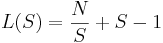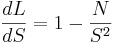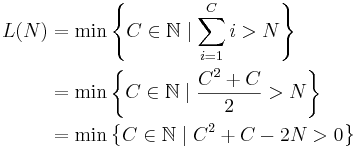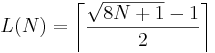## Interview Questions: Two Bowling Balls

by Jesse Farmer on Tuesday, April 15, 2008

This post is the first in a series I'm calling "interview questions," where I discuss interview questions I've been handed in my time out here in the Bay Area. Since I'm an engineer by trade most of the questions relate directly to technical topics. I'll also cover general interview strategies and advice — probably by serving myself up as an example of what not to do in an interview.

I know people keep a repertoire of interview questions at hand, so I'm not going to name names when discussing the questions. Anyhow, let's get started!

### The Question

You're standing in front of a 100 story building with two identical bowling balls. You've been tasked with testing the bowling balls' resilience. The building has a stairwell with a window at each story from which you can (conveniently) drop bowling balls.

To test the bowling balls you need to find the first floor at which they break. It might be the 100th floor or it might be the 50th floor, but if it breaks somewhere in the middle you know it will break at every floor above.

Devise an algorithm which guarantees you'll find the first floor at which one of your bowling balls will break. You're graded on your algorithm's worst-case running time.

### A Few Preliminaries

The original problem stated that the building had 100 floors, but it may as well have N floors. Using N rather than 100 will make it easier to quantify the performance of the algorithm, so that's what I'm going to do.

### Solution 1: The Naïve Solution

Ok, there's one blindingly obvious solution: take one of the bowling balls and drop it from every floor, starting from the first. At worst this will take N tries, where N is the number of stories on the building.

Interview Advice:: In an actual interview situation don't be afraid to say the obvious solution, even if you know there's a better one. Problem solving is iterative and your answer should be, too.

### Solution 2: Two Bowling Balls

We know the first solution is probably sub-optimal because it doesn't make use of both bowling balls. To give us some ideas let's just pick a floor, say the 50th floor, and drop one of the balls — we has nothing to lose since we know we can do it with only one.

If our building is 100 floors and we dropped one of the balls from the 50th floor one of two thing will happen: the ball will either break or it won't. If it breaks then we know the floor we're looking for is somewhere between floors 1-49. If it doesn't then we know it's somewhere between floors 51-100. In either case we've halved the size of the search space and now need at most N/2 (or 50) tries.

But 50 was arbitrary. What about other numbers? What happens if we drop the ball on the third floor? If it breaks then we can use the second ball to test floors 1-2, taking at most 3 tries. If it doesn't break then we try the same experiment again, dropping the ball from another floor.

Here's one possible strategy: pick a number S and call it the skip number. We drop one ball every S floors until it breaks on the kth try. We then use the second ball to try every floor between floors (k-1)*S and k*S.

As an example, let N=100 and S=4. We'd try floors 4,8,12,16,... with one bowling ball until it breaks. Let's say it breaks on the 60th floor. Since it didn't break on the 56th floor we know the culprit is somewhere on floor 57, 58, 59, and we can use the second ball to test those floor one at a time using the naÃ¯ve strategy.

What is the best skip size? Obviously S=100 isn't ideal since that is equivalent to the naÃ¯ve strategy, as is S=1. But we know both S=50 and S=4 are better, so there must be an optimal strategy somewhere between. To find this strategy let L(S) be the number of drops requires in the worst-case scenario for a skip number of S. If you work it out you'll getWe want to minimize this function. Bringing back our high school calculus, the derivative of L(S) isSetting the derivative equal to zero impliesFor N=100 this gives an optima skip of S=10. If N isn't a perfect square you'll have to work out which skip gives the "correct" solution.

### Solution 3: You can do better...

At this point in the interview you're probably pretty happy with yourself. The above took you a few minutes to work out, perhaps with some prodding by the interviewer. But then you hear that dreaded question, "Can you do any better?"

The interviewer isn't a jackass, though, and gives you a hint. He points out that it seems like we should be able to find a solution that works equally well irrespective of where the bad floor is. That is, it should take the same number of turns if its on the 100th floor as it would if it were on a lower floor.

We have a baseline for ourselves. For N=100 and S=10 we know we can do it in at most 19 turns. This can act as a sort of counter — if we beat this number at every step we've come up with a strictly better algorithm. So, at every step, we want to be able to find the floor in question in no more than 18 steps.

Let's start by dropping the first ball on the 18th floor. If it breaks we can test floors 1-17 with the second ball, taking at most 18 turns. If it doesn't break, we've used up one of our turns, leaving us with 17 turns left.

So, the next floor we should test is 18+17, or the 35th floor. If it breaks we can test floors 19-35, taking at most 18 turns. We can continue this way, shrinking the step size by one each time. Now we know we can do it in at least 18 steps.

But why not 17? If we repeat the above steps, starting with a counter of 17 rather than 18, we get an algorithm that takes at most 16 steps. Then, using 16 as a counter, we get an algorithm that takes at most 15 steps. We can't do this forever, since there's no possible algorithm that takes at most one step. So where is the end of the line?

The problem is that for this algorithm to work the first ball needs to be able to skip one fewer each time and still cover all 100 floors. If we set our counter to C that means we must have 1+2+...+C > 100.

Here's the math:Using the quadratic formula to find the exact solution and then taking into account the fact that we want an integer solution givesas the worst possible case for our third strategy. L(100) = 14, which checks out.

That's the best solution I know, and it was the best solution the interviewer knew, too. Can you do any better?

### After The Interview

This was one of a few questions I was asked by one of four interviewers. I worked through the problem above, basically as it was written out, albeit with more digressions. How did the interview wind up going? I wasn't offered a job. At least I got a good interview question out of it, though.